# Abelian category

(diff) ← Older revision | Latest revision (diff) | Newer revision → (diff)

A category that displays some of the characteristic properties of the category of all Abelian groups. Abelian categories were introduced as the basis for an abstract construction of homological algebra . A categoryis said to be Abelian  if it satisfies the following axioms:

A0. A null object exists (cf. Null object of a category).

A1. Each morphism has a kernel (cf. Kernel of a morphism in a category) and a cokernel.

A2. Each monomorphism is a normal monomorphism, so that it occurs as the kernel of a morphism; each epimorphism is a normal epimorphism.

A3. A product and a coproduct exist for each pair of objects (cf. Product of a family of objects in a category).

In defining an Abelian category it is often assumed thatis a locally small category (cf. Small category). The coproduct of two objectsandof an Abelian category is also know as the direct sum of these objects and is denoted by,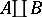or.

## Contents

### Examples of Abelian categories.

1) The dual category of an Abelian category is also an Abelian category.

2) The category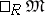of all unitary left modules over an arbitrary associative ringwith a unit element and all-module homomorphisms is an Abelian category (e.g. the category of all Abelian groups).

3) Any full subcategory of an Abelian category which contains for each one of its morphisms also the kernel and cokernel of that morphism, and which contains for each pair of objectsandalso their product and coproduct, is an Abelian category.

The small Abelian categories are exhausted by the subcategories of the above type of categoriesof unitary left modules. Accordingly, the following Mitchell theorem is valid: For each small Abelian category there exists a full exact imbedding into some category.

4) Any category of diagrams, with diagram scheme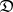over an Abelian category, is an Abelian category. In the schemeone may distinguish the set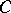of commutativity relations, i.e. the set of pairs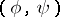of paths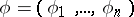,inwith a common begin and end. Then the complete subcategory of the categorygenerated by all those diagrams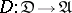that satisfyis an Abelian category. In particular, ifis a small category and if the setconsists of all pairs of the formwith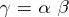, then the corresponding subcategory is the Abelian category of one-place covariant functors frominto(cf. Functor).

Suppose that a null object exists in a small category. A functor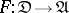will then be called normalized if it takes a null object into a null object. The complete subcategory of the category of functors generated by the normalized functors will then be an Abelian category. In particular, ifis a category the objects of which are all integers plus the null object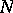, while the non-null non-identity morphisms form a sequence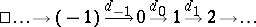in which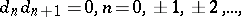then the corresponding subcategory generated by the normalized functors is called the category of complexes over. On the category of complexes there are defined the additive functors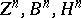of-dimensional cycles,-dimensional boundaries and-dimensional homologies, respectively, with values in. These constitute the basis for the development of homological algebra.

5) A full subcategoryof an Abelian categoryis said to be dense if it contains all subobjects (cf. Subobject) and quotient objects (cf. Quotient object) of its objects, and if for the exact sequence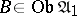if and only if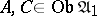. The quotient categoryis then constructed as follows. Let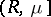be a subobject of the direct sumwith projectionsand let the square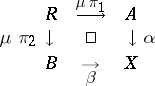be co-universal (i.e. a co-fibred product). The subobjectis called an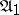-subobject if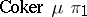,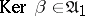. Two-subobjects are equivalent if they contain some-subobject. By definition, the set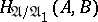consists of the equivalence classes of-subobjects. The usual multiplication of binary relations is compatible with the equivalence relation thus introduced, which makes it possible to construct the quotient category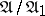, which is an Abelian category. The exact functor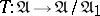is defined by assigning to each morphism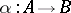its graph in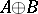. A subcategoryis said to be a localizing subcategory ifhas a complete univalent right-adjoint functor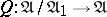.

6) For any topological spacethe category of left-modules over, whereis a sheaf of rings with unit element over, is an Abelian category.

It is possible to introduce into any Abelian categorya partial sum of morphisms so thatbecomes an additive category. For this reason the product and the coproduct of any pair of objects in an Abelian category are identical. Moreover, in defining an Abelian category it suffices to assume the existence of either products or coproducts. Any Abelian category is a bicategory with a unique bicategorical structure. These properties characterize an Abelian category: A categorywith finite products is Abelian if and only if it is additive and if any morphismhas a kernel and a cokernel and can be decomposed into a productin whichis an isomorphism.

The Mitchell theorem quoted above constitutes the underlying principle of the so-called "diagram-chasing" method in an Abelian category: Any proposition about commutative diagrams that is valid for all categories of left modulesand that is a consequence of the exactness of certain sequences of morphisms, is valid in all Abelian categories.

In a locally small Abelian category, the-subobjects of an arbitrary object form a Dedekind lattice. If products (or coproducts) of any family of objects exist in, this lattice will be complete. These conditions are known to be met if there is a generating objectinand if the coproducts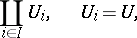exist for any set. These conditions are satisfied, for instance, by Grothendieck categories (cf. Grothendieck category), which are equivalent to the quotient categories of the category of modules by a localizing subcategory (the Gabriel–Popescu theorem).

How to Cite This Entry:
Abelian category. Encyclopedia of Mathematics. URL: http://encyclopediaofmath.org/index.php?title=Abelian_category&oldid=17350
This article was adapted from an original article by M.Sh. Tsalenko (originator), which appeared in Encyclopedia of Mathematics - ISBN 1402006098. See original article